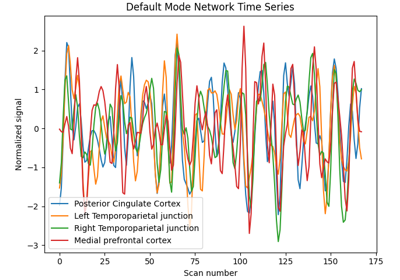Note

This page is a reference documentation. It only explains the function signature, and not how to use it. Please refer to the user guide for the big picture.

# nilearn.plotting.plot_markers#

nilearn.plotting.plot_markers(node_values, node_coords, node_size='auto', node_cmap=<matplotlib.colors.ListedColormap object>, node_vmin=None, node_vmax=None, node_threshold=None, alpha=0.7, output_file=None, display_mode='ortho', figure=None, axes=None, title=None, annotate=True, black_bg=False, node_kwargs=None, colorbar=True)[source]#

Plot network nodes (markers) on top of the brain glass schematics.

Nodes are color coded according to provided nodal measure. Nodal measure usually represents some notion of node importance.

Parameters:
node_valuesarray_like of length n

Vector containing nodal importance measure. Each node will be colored according to corresponding node value.

node_coordsnumpy array_like of shape (n, 3)

3d coordinates of the graph nodes in world space.

node_size‘auto’ or scalar or array-like, optional

Size(s) of the nodes in points^2. By default the size of the node is inversely proportional to the number of nodes.

node_cmapstr or colormap, optional

Colormap used to represent the node measure. Default=plt.cm.viridis_r.

node_vminfloat, optional

Lower bound of the colormap. If None, the min of the node_values is used.

node_vmaxfloat, optional

Upper bound of the colormap. If None, the min of the node_values is used.

node_thresholdfloat

If provided only the nodes with a value greater than node_threshold will be shown.

alphafloat between 0 and 1, optional

Alpha transparency for markers. Default=0.7.

output_file`str`, or None, optional

The name of an image file to export the plot to. Valid extensions are .png, .pdf, .svg. If `output_file` is not None, the plot is saved to a file, and the display is closed.

display_modestring, optional

Choose the direction of the cuts: ‘x’ - sagittal, ‘y’ - coronal, ‘z’ - axial, ‘l’ - sagittal left hemisphere only, ‘r’ - sagittal right hemisphere only, ‘ortho’ - three cuts are performed in orthogonal directions. Possible values are: ‘ortho’, ‘x’, ‘y’, ‘z’, ‘xz’, ‘yx’, ‘yz’, ‘l’, ‘r’, ‘lr’, ‘lzr’, ‘lyr’, ‘lzry’, ‘lyrz’. Default=’ortho’.

figure`int`, or `matplotlib.figure.Figure`, or None, optional

Matplotlib figure used or its number. If `None` is given, a new figure is created.

axes`matplotlib.axes.Axes`, or 4 tupleof `float`: (xmin, ymin, width, height), optional

The axes, or the coordinates, in matplotlib figure space, of the axes used to display the plot. If `None`, the complete figure is used.

title`str`, or None, optional

The title displayed on the figure. Default=None.

annotate`bool`, optional

If `annotate` is `True`, positions and left/right annotation are added to the plot. Default=True.

black_bg`bool`, or ‘auto’, optional

If `True`, the background of the image is set to be black. If you wish to save figures with a black background, you will need to pass facecolor=’k’, edgecolor=’k’ to `matplotlib.pyplot.savefig`. Default=False.

node_kwargsdict, optional

will be passed as kwargs to the plt.scatter call that plots all the nodes in one go

colorbar`bool`, optional

If `True`, display a colorbar on the right of the plots. Default=True.

## Examples using `nilearn.plotting.plot_markers`#Extract signals on spheres and plot a connectome

Extract signals on spheres and plot a connectome